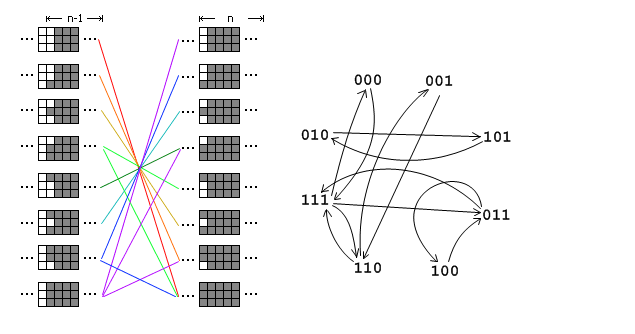## 2019 ICPC南昌邀请赛网络赛 K MORE XOR

(⊙﹏⊙)不知道为什么比赛时候这个题和D题死推不出来，比赛完了5分钟ac，我大概脑子坏掉了。真的是超超超超级简单的一个题，但是我光顾着用数学公式推了所以推出来结果巨复杂(⊙﹏⊙)服了自己了。

$g(l,r)= \begin{cases} 0& \text{(r-l+1) is odd}\\ p2[l-2]\oplus p2[r]& \text{else } \end{cases}$

$w(l,r)= \begin{cases} 0& \text{t=0}\\ p31[r]\oplus p31[l-3]& \text{t=1 and r is odd}\\ p32[r]\oplus p32[l-3]& \text{t=1 and r is even}\\ p3[l-3]\oplus p3[r]& \text{t=2}\\ p32[r-1]\oplus p32[l-2]& \text{t=3 and r is odd}\\ p31[r]\oplus p31[l-3]& \text{t=3 and r is even} \end{cases}$

## [组合数学][数论][矩阵][优化][Polya][状压DP][x] XDOJ 1296 敬老师的手环II## [数论][二次剩余应用][组合数][等比数列] ZOJ 3774 Power of Fibonacci继续阅读“[数论][二次剩余应用][组合数][等比数列] ZOJ 3774 Power of Fibonacci”

（复习期末期间日常的小题

## [二维DP][组合计数][利用对称性简化问题] UVALive 3295

https://vjudge.net/problem/UVALive-3295

wa的话有一个问题是，在gcd的算法中如果a和b有一个数字为0那么返回值就会是0，这种情况在这里的递推过程中是会出现问题的，需要注意让循环从2开始，也算是一个小小的坑吧。。以前没有注意到。